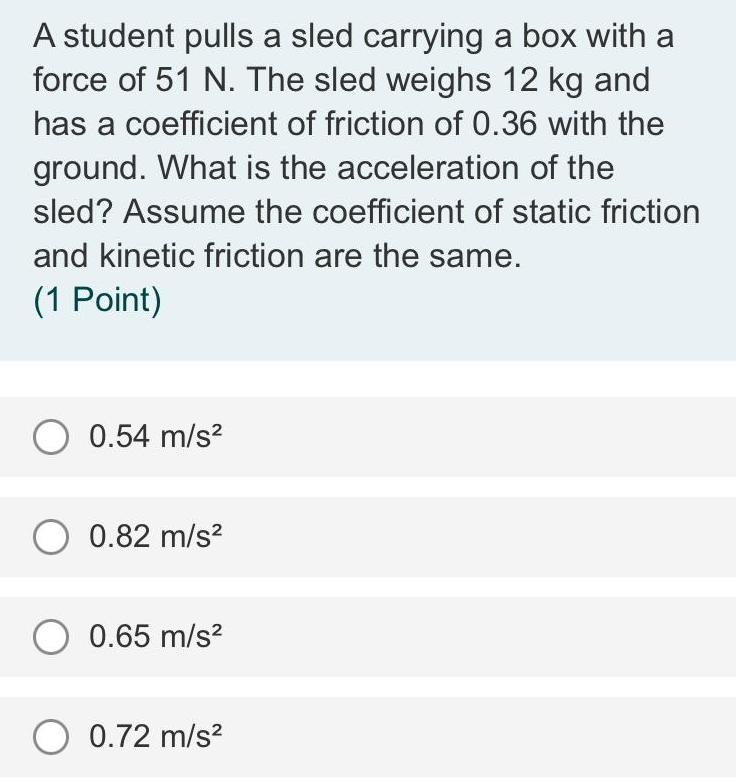Question:

# A student pulls a sled carrying a box with a force of 51 N.

Last updated: 8/3/2022A student pulls a sled carrying a box with a force of 51 N. The sled weighs 12 kg and has a coefficient of friction of 0.36 with the ground. What is the acceleration of the sled? Assume the coefficient of static friction and kinetic friction are the same. 0.54 m/s² 0.82 m/s² 0.65 m/s² 0.72 m/s²